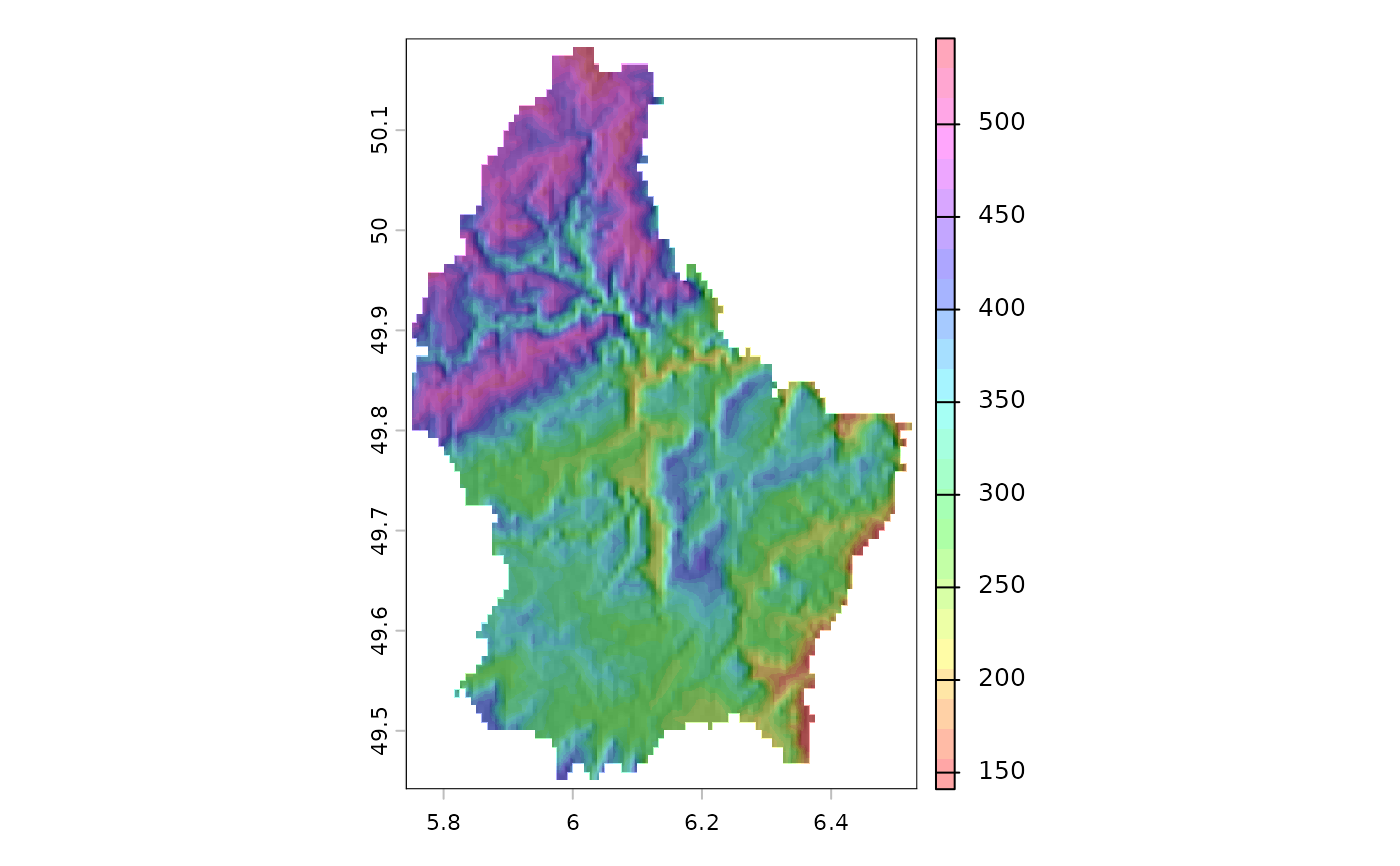Compute hill shade from slope and aspect layers (both in radians). Slope and aspect can be computed with function terrain.

A hill shade layer is often used as a backdrop on top of which another, semi-transparent, layer is drawn.

shade(slope, aspect, angle=45, direction=0, normalize=FALSE,
filename="", overwrite=FALSE, ...)

## Arguments

slope

aspect

SpatRaster with aspect values (in radians)

angle

The elevation angle(s) of the light source (sun), in degrees

direction

The direction (azimuth) angle(s) of the light source (sun), in degrees

normalize

Logical. If TRUE, values below zero are set to zero and the results are multiplied with 255

filename

character. Output filename

overwrite

logical. If TRUE, filename is overwritten

...

additional arguments for writing files as in writeRaster

terrain

## Examples

f <- system.file("ex/elev.tif", package="terra")
r <- rast(f)
alt <- disagg(r, 10, method="bilinear")